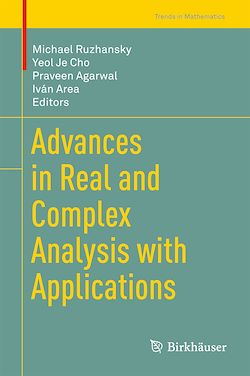301 Pages
English

# Advances in Real and Complex Analysis with Applications

-Description

This book discusses a variety of topics in mathematics and engineering as well as their applications, clearly explaining the mathematical concepts in the simplest possible way and illustrating them with a number of solved examples. The topics include real and complex analysis, special functions and analytic number theory, q-series, Ramanujan’s mathematics, fractional calculus, Clifford and harmonic analysis, graph theory, complex analysis, complex dynamical systems, complex function spaces and operator theory, geometric analysis of complex manifolds, geometric function theory, Riemannian surfaces, Teichmüller spaces and Kleinian groups, engineering applications of complex analytic methods, nonlinear analysis, inequality theory, potential theory, partial differential equations, numerical analysis , fixed-point theory, variational inequality, equilibrium problems, optimization problems, stability of functional equations, and mathematical physics.

It includes papers presented at the 24th International Conference on Finite or Infinite Dimensional Complex Analysis and Applications (24ICFIDCAA), held at the Anand International College of Engineering, Jaipur, 22–26 August 2016. The book is a valuable resource for researchers in real and complex analysis.

Subjects

##### Mathematical analysis

Informations